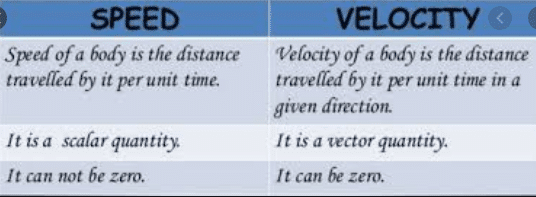Mechanics

# Velocity: Definition, Formula and Examples

In this post, you’ll learn Examples of Velocity in everyday life. If you want to learn about Velocity in detail, then you are at the Right Place.
Let’s Dive right in:
Contents:

• Velocity Definition
• Velocity Formula
• Velocity Unit
• Examples
• Much more

## What is Velocity?

Velocity is defined as: “The rate of displacement of a body is called its velocity.”The velocity tells us not only the speed of a body but also the direction along which the body is moving. The velocity of a body is a vector quantity. It is equal to the displacement of a body in unit time. And is represented as:

Velocity=displacement/time
V=d/t

SI unit of velocity is the same as speed i.e., meter per second.

### Uniform velocity

“A body has uniform velocity if it covers equal displacement in equal intervals of time however short the interval may be.”In equation( d=v t ) is the average velocity of a body during time t. It is because the velocity of the body may be changing during the time interval. However, in many cases, the speed and direction of a body do not change. In such a case the body possesses uniform velocity. That is the velocity of a body during any interval of time has the same magnitude and direction.

Average velocity is the total displacement covered in any direction in a total interval of time.” or ratio of total displacement to time.

### average velocity formula

Vav = (final velocity + initial velocity)/2

## Difference between speed and velocity in physics